# Mathematics Paper 2 Questions and Answers - Form 4 Term 1 Opener Exams 2023

INSTRUCTION.
• Answer all questions in the spaces provided.
1. Factorize the expression 2x2 + x – 15. Hence solve the equation 2x2 + x − 15 = 0. (3mks)
2. Two people each working for 8 hours a day can cultivate an acre of land in 4 days. How long would 6 people, each working 4 hours a day, take to cultivate 4 acres? (3mks)
3. In the figure below, O is the centre of the circle <POQ = (π/2)c and PQ=24cm.1.
1. Express <POQ in degrees. (1mk)
2. Find the radius r of the circle. (1mk)
2. Find the area of the shaded segment. (2mks)
4. The difference between the eight term and the fourth term of an AP is 24. The first term of this series exceeds the common difference by 4. Find the tenth term of the series. (3mks)
5. Simplify √3 − √2  , giving your answer in the form a + b√c, where a,b and c are real numbers. (3mks)
√3 + √2
6. Find the first five terms of the expansion (2−1/x)8, hence, evaluate (1.75)8 (3mks)
7. A quantity p is partly constant and partly varies as the square of Q. when Q=2,p=40 and when Q=3, P=65. Determine the value of p when Q=4. (3mks)
8. Given that x =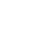and y=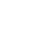Find:
1. XY (2mks)
2. (XY)-1 (2mks)
9. Construct the triangle XYZ in which XY = 5cm, <YXZ = 60° and <XYZ = 90°. Measure YZ, hence use area = ½ absinθ to, calculate the area of triangle XYZ. (3mks)
10. The position vectors of points P,Q and R are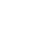and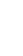respectively.
1. Find
1. PQ (1mk)
2. PR (1mk)
2. Hence or otherwise, show that points P,Q and R are collinear. (2mks)
11. In an experiment involving two variables E and C, the following results were obtained.
 E 4 7 10 13 16 19 C 15.1 20.2 23.9 27.3 30.1 33.1
1. On the grid provided below plot the values of C against E hence use the plotted points to draw the line of best fit. (2mks)
2. Hence determine the equation connecting E and C. (2mks)
12. A and B are two points on the surface of the earth. Their position lie on the equator such that A (0°,30°W) and B(0°,60°E). calculate:
1. The distance in nautical miles between A and B. (2mks)
2. The time difference between A and B. (2mks)
13. Find the value of y in the equation: (2mks)
Log7 (1/343)    =  y
14. Mwanyumba invested some money in a housing finance company that offered compound interest of 2% per annum. After 3 years, her money had accumulated to sh 106120.80. How much money had she invested? (3mks)
15. Find the gradient of y = x2 − 3x at the point (2, −2) (2mks)
16. An object starts from rest and its velocity is measured every second for 6 seconds as shown in the table below.
 Time(t) 0 1 2 3 4 5 6 Velocity v(ms−1) 0 12 24 35 41 45 47
Use the trapezium rule to calculate the area between t= 1 and t=6 seconds. (2mks)

SECTION II (50 marks)

1. The table below shows the income tax rates for a certain year.
 Monthly taxable income in Ksh. Tax rate (%) in each shilling 1 − 11180 10 11181 − 21714 15 21715 − 32248 20 32249 – 42782 25 Over 42782 30
1.  During the year, Obonyo’s monthly income was as follows;
Basic salary                               Ksh 40,000
House allowance                       Ksh 11,090
Commuter allowance                 Ksh   7,000
Calculate;
1. Obonyo’s total monthy taxable income. (2mks)
2. The total income tax charged on Obonyo’s monthly income. (6mks)
2. Obonyo’s net monthly tax was Ksh 10,750.80. Determine the monthly tax relief allowed. (2mks)
2. The figure below represents a cross-section of a concrete prism whose length is 3m.
Given that AB=FE and AGF is a semi circle.1. Calculate the total surface area of the prism. (5mks)
2. Determine the volume of the concrete used to make the prism. (3mks)
3. Given that 1cm3 of concrete weighs 6.8 grams, find the mass of the prism in kg. (2mks)
3. Wamuyu purchased (2x−1) identical pens for ksh. 180. Nyambura purchased (3x+1) identical pencils for ksh. 200.
1. Write an expression for the;
1. Price of one pen. (1mk)
2. Price of one pencil (1mk)
2. A pen costs Ksh 4 more than a pencil. Form an equation to represent the information above and hence solve for x. (4mks)
3. Later the price of a pen went up by 25% while that of a pencil remained unchanged. A school bought 30 pencils and 16 pens. Determine the total amount of money spent by the school. (4mks)
4. The first term of an arithmetic sequence is 2. The first term of a geometric sequence is also 2. The common ratio of geometric sequence equals the common differences of the arithmetic sequence. By taking d as the common difference and r as the common ratio.
1. Write an expression connecting d and r. (1mk)
2. The third term of the geometric sequence exceeds the square of the first term of the arithmetic sequence by 124. Find:
1. The common ratio (3mks)
2. The common difference (1mk)
3. Determine;
1. The first ten terms of the arithmetic sequence. (2mks)
2. Hence or otherwise, find the sum of the first 10 terms of the arithmetic sequence. (3mks)
5.
1. Given that y= x3 + 2x2 − 5x − 8, complete the table below; (2mks)
 X −4 −3 −2 −1 0 1 2 3 X3 −64 −27 −8 −1 0 1 8 27 2x2 32 18 8 2 0 2 8 18 −5x 20 15 10 5 0 −5 −10 −15 −8 −8 −8 −8 −8 −8 −8 −8 −8 Y
2. On the grid provided below, using a suitable scale draw the graph of y = x3 + 2x2 − 5x − 8 for the values of x in the range −4 ≤ x ≤ 3. (3mks)
3. Use your graph to solve the equations. (2mks)
x3 + 2x2 − 5x − 8 = 0
4. By drawing a straight line, use the graph to solve the equation –x3 − 2x2 + 3x + 3 = 0 (3mks)
6. Alberto LUCCI is an Italian tourist who regularly visits Masai Mara while on tour in Kenya.  The probability that Alberto goes to Maasai Mara is ¼ , if he visits Maasai Mara, the probability that he sees a wilde beest is ½. If he does not go to Maasai Mara, the probability that he will see a wilde beest is 1/3
1. Draw a tree diagram to show the above outcome. (2mks)
2. Find the probability that Alberto will:
1. Visit Maasai Mara and see a wilde beest. (2mks)
2. Not visit Maasai Mara and see a wilde beest. (2mks)
3. See a wilde beest. (3mks)
7. In the figure below C is a point of AB such that AC:CB=3:1 and D is the mid-point of OA. OC and BD intersect at X.Given that OA = a and OB = b
1. Write the vectors below in terms of a and b.
1. AB (1mk)
2. OC (2mks)
3. BD (1mk)
2. Given that BX = hBD, express OX in terms of a,b and h. (1mk)
3. If OX = kOC, find the values of h and k. (4mks)
4. Hence write the ratio OX:XC (1mk)
8. A particle moves in a straight line in such a way that its distance s metres from a fixed point O after t seconds is given by
S =1t33t2 + 2t
3       2
1. Find the position of the particle when it is momentarily at rest. (5mks)
2. Calculate the acceleration of the particle at this time when it is momentarily at rest. (2mks)
3. Find the maximum or minimum velocity of the particle. (3mks)## MARKING SCHEME

1. Factorize the expression 2x2 + x – 15. Hence solve the equation 2x2 + x − 15 = 0. (3mks)
(6, −5)
2x2 + 6x − 5x −15
2x(x+3) − 5(x+3)
(2x−5)(x+3)
(2x−5)(x+3) = 0
2x − 5 = 0
∴ x = 2½ or x + 3 = 0
x = −3
2. Two people each working for 8 hours a day can cultivate an acre of land in 4 days. How long would 6 people, each working 4 hours a day, take to cultivate 4 acres? (3mks)2:6
8:4
4:1
2/6 × 8/4 × 4/× 4
= 102/3days
3. In the figure below, O is the centre of the circle <POQ = (π/2)c and PQ=24cm.1.
1. Express <POQ in degrees. (1mk)
c → 360°
(π/2)c → ?
π/2 × 1/ × 360
= 90°
2. Find the radius r of the circle. (1mk)
r² + r² = 24²
2r² = 576
r = √288
= 16.97
2. Find the area of the shaded segment. (2mks)
A = θ/360πr² − ½ab sin C
A = 90/360 × π × 16.97− ½ × 16.97² sin 90
= 226.3 − 143.99
= 82.31cm²
4. The difference between the eight term and the fourth term of an AP is 24. The first term of this series exceeds the common difference by 4. Find the tenth term of the series. (3mks)
Let 8th = a + 7d
∴ 4th = a + 3d
a + 7d − (a + 3d) = 24
∴ 4d = 24
d = 6
a − d = 4
a − 6 = 4
∴ a= 10
10th = a + 9d
= 10 + (9×6)
= 64
5. Simplify √3 − √2  , giving your answer in the form a + b√c, where a,b and c are real numbers. (3mks)
√3 + √2
√3 − √2   − √3 − √2
√3 + √2      √3 − √2
Num
√3.√3 − √3.√2 − √3.√2 + √2.√2
3 − 2√6 + 2
5 − 2√6
Den.
√3.√3 − √3.√2 + √3.√2 − √2.√2
3 − 2
= 1
5 − 2√6
1
= 5 − 2√6
6. Find the first five terms of the expansion (2−1/x)8, hence, evaluate (1.75)8 (3mks)7. A quantity p is partly constant and partly varies as the square of Q. when Q=2,p=40 and when Q=3, P=65. Determine the value of p when Q=4. (3mks)
P = K + CQ2
(i) 40 = K + 4C
(ii) 65 = K + 9C
(ii) − (i)
25 = 5C
∴ C = 5
K = 20
⇒ P = 20 + 5Q²
When Q = 4
P = 20 + 5 × 42
= 100
8. Given that x =and y=Find:
1. XY (2mks)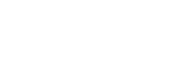2. (XY)-1 (2mks)9. Construct the triangle XYZ in which XY = 5cm, <YXZ = 60° and <XYZ = 90°. Measure YZ, hence use area = ½ absinθ to, calculate the area of triangle XYZ. (3mks)YZ = 10.2 ± 1cm
Area =  ½ × 10.2 × 5 Sin 60
= 5.1 × 5 × 0.867
= 22.1cm²
10. The position vectors of points P,Q and R areandrespectively.
1. Find
1. PQ (1mk)
PQ = Q − P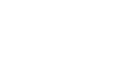2. PR (1mk)
PR = R − P2. Hence or otherwise, show that points P,Q and R are collinear. (2mks)∴ ½PQ = 1/5 PR
PQ = 2/5PR and P is common
11. In an experiment involving two variables E and C, the following results were obtained.
 E 4 7 10 13 16 19 C 15.1 20.2 23.9 27.3 30.1 33.1
1. On the grid provided below plot the values of C against E hence use the plotted points to draw the line of best fit. (2mks)2. Hence determine the equation connecting E and C. (2mks)
19 − 16
= 3/3 = 1
L Intercept = 14.1
⇒ C = 1 × E + 14.1
C = E + 14.1
12. A and B are two points on the surface of the earth. Their position lie on the equator such that A (0°,30°W) and B(0°,60°E). calculate:
1. The distance in nautical miles between A and B. (2mks)
Let α = 30+ 60
= 90°
∴ AB = 60 × 90
= 5400nm
2. The time difference between A and B. (2mks)
1° → 4'
90° → ?
90 × 4 = 360'
360 ÷ 60
= 6 hrs.
13. Find the value of y in the equation: (2mks)
Log7 (1/343)    =  y
1/343    =  7y
7−3 = 7y
∴ y = − 3
14. Mwanyumba invested some money in a housing finance company that offered compound interest of 2% per annum. After 3 years, her money had accumulated to sh 106120.80. How much money had she invested? (3mks)
A = Sh. 106120.80
P = ?
r = 2%
n = 3
A = P(1 + r/100)n
106120.80 = P(1 + 2/100)3
∴ P = 106120.80
1.061208
= Sh 100,000
15. Find the gradient of y = x2 − 3x at the point (2, −2) (2mks)
dy/dx = 2x − 3
at x = 2
Gradient = 2 × 2 − 3
= 1
16. An object starts from rest and its velocity is measured every second for 6 seconds as shown in the table below.
 Time(t) 0 1 2 3 4 5 6 Velocity v(ms−1) 0 12 24 35 41 45 47 y0 y1 y2 y3 y4 yn
Use the trapezium rule to calculate the area between t= 1 and t=6 seconds. (2mks)
h = 1
½ × 1 { 12 + 47 + 2(24 + 35 + 41 + 45)}
½ × {59 + 290}
= 174.5 sq. units

SECTION II (50 marks)

1. The table below shows the income tax rates for a certain year.
 Monthly taxable income in Ksh. Tax rate (%) in each shilling 1 − 11180 10 11181 − 21714 15 21715 − 32248 20 32249 – 42782 25 Over 42782 30
1.  During the year, Obonyo’s monthly income was as follows;
Basic salary                               Ksh 40,000
House allowance                       Ksh 11,090
Commuter allowance                 Ksh   7,000
Calculate;
1. Obonyo’s total monthy taxable income. (2mks)
40000 + 11090 + 7000
= Ksh. 58090
2. The total income tax charged on Obonyo’s monthly income. (6mks)
1st slab 11180 × 10/100 = 1118
2nd "     10534 × 15/100 = 1580.1
3rd "      10534 × 20/100 = 2106.8
4th "      10534 × 25/100 = 2633.5
5th "       15308 × 30/100 = 4592.4
Total tax = 1118 + 1580.1 + 2106.8 + 2633.5 + 4592.4
= 12030.80
2. Obonyo’s net monthly tax was Ksh 10,750.80. Determine the monthly tax relief allowed. (2mks)
Relief = 12030.80 − 10750.80
= Ksh 1280
2. The figure below represents a cross-section of a concrete prism whose length is 3m.
Given that AB=FE and AGF is a semi circle.1. Calculate the total surface area of the prism. (5mks)
Diameter Af = 24 − (6×2) = 12cm
∴AGF = πD = 6π = 18.85cm
2
Curved S. A = 18.85 × 300 = 5654.87cm²
Cross section area = (24 × 18 − ½π × 6²) × 2
= (432 − 56055) × 2
= 750.9cm²
Base area = 24 × 300 = 7200cm²
Side faces = (18×300) × 2
= 10,800cm²
Top faces = 6 × 300 × 2
= 3600cm²
Total = 5654.87
750.9
7200
10800
+ 3600
=  28005.77cm²
2. Determine the volume of the concrete used to make the prism. (3mks)
V = Cross section area × Length
= 750.9 × 300cm³
= 225270cm³
3. Given that 1cm3 of concrete weighs 6.8 grams, find the mass of the prism in kg. (2mks)
1cm³ → 6.8g
225270cm³ → ?
225270 × 6.8
1000
= 1531.836kg
3. Wamuyu purchased (2x−1) identical pens for ksh. 180. Nyambura purchased (3x+1) identical pencils for ksh. 200.
1. Write an expression for the;
1. Price of one pen. (1mk)
180
(2x − 1)
2. Price of one pencil (1mk)
200
(3x + 1)
2. A pen costs Ksh 4 more than a pencil. Form an equation to represent the information above and hence solve for x. (4mks)
200    + 4 =    180
(3x + 1)         (2x − 1)
200 + 12x + 4  180
3x + 1
2x − 1
(204 + 12x)(2x−1) = 180(3x+1)
24x² − 144x − 384 = 0
x² − 6x − 16 = 0
(x − 8)(x + 2) = 0
x = 8 or x = −2
x = 8
3. Later the price of a pen went up by 25% while that of a pencil remained unchanged. A school bought 30 pencils and 16 pens. Determine the total amount of money spent by the school. (4mks)
∴ Price of pen =      180
(2×8 − 1)
= Sh.12
Price of pencil =
200
(3×8 + 1)
= Sh. 8
New Pen's price = 125/100 × 12
= Sh.15
⇒ 30 × 8 + 16 × 15
240 + 240+
=Sh. 480
4. The first term of an arithmetic sequence is 2. The first term of a geometric sequence is also 2. The common ratio of geometric sequence equals the common differences of the arithmetic sequence. By taking d as the common difference and r as the common ratio.
1. Write an expression connecting d and r. (1mk)
d = r
2. The third term of the geometric sequence exceeds the square of the first term of the arithmetic sequence by 124. Find:
1. The common ratio (3mks)
3rd term = ar²
= 2r²
2r² − 2² = 124
2r² = 128
r² = 64
∴ r = ± 8
2. The common difference (1mk)
d = r
∴ d = ± 8
3. Determine;
1. The first ten terms of the arithmetic sequence. (2mks)
When d = 8     2, 10, 18, 26, 34, 42, 50, 58, 64, 72
When d = −8   2, −6, −14, −22, −30, −38, −46, −54, −62, −70
2. Hence or otherwise, find the sum of the first 10 terms of the arithmetic sequence. (3mks)
If d = 8
S10 = 10/2{2 × 2 + 9 × 8}
=5(4 + 72)
= 5 × 76
= 380
If d = −8
S10 = 10/2 { 2 × 2 + 9 × −8}
= 5{4 − 72}
= − 340
5.
1. Given that y= x3 + 2x2 − 5x − 8, complete the table below; (2mks)
 X −4 −3 −2 −1 0 1 2 3 X3 −64 −27 −8 −1 0 1 8 27 2x2 32 18 8 2 0 2 8 18 −5x 20 15 10 5 0 −5 −10 −15 −8 −8 −8 −8 −8 −8 −8 −8 −8 Y −20 −2 2 −2 −8 −10 −2 22
2. On the grid provided below, using a suitable scale draw the graph of y = x3 + 2x2 − 5x − 8 for the values of x in the range −4 ≤ x ≤ 3. (3mks)3. Use your graph to solve the equations. (2mks)
x3 + 2x2 − 5x − 8 = 0
Line y = 0
Solutions x = −2.8 or x = −1.3 or x = 2.1
4. By drawing a straight line, use the graph to solve the equation –x3 − 2x2 + 3x + 3 = 0 (3mks)
y =   x3 + 2x2 − 5x − 8
0 = −x3 + 2x2 + 3x + 3
Line y =      −2x − 5
y = −2x−5
Solutions x = −2.7 or x = −0.8 or x =1.5
6. Alberto LUCCI is an Italian tourist who regularly visits Masai Mara while on tour in Kenya.  The probability that Alberto goes to Maasai Mara is ¼ , if he visits Maasai Mara, the probability that he sees a wilde beest is ½. If he does not go to Maasai Mara, the probability that he will see a wilde beest is 1/3
1. Draw a tree diagram to show the above outcome. (2mks)2. Find the probability that Alberto will:
1. Visit Maasai Mara and see a wilde beest. (2mks)
P(MW) = ¼ × ½ = 1/8
2. Not visit Maasai Mara and see a wilde beest. (2mks)
P(M'W) = 3/4 × 1/3
=¼
3. See a wilde beest. (3mks)
P(MW) or P(M'W)
¼ × ½ + 3/4 × 1/3
1/8 + ¼
= 3/8
7. In the figure below C is a point of AB such that AC:CB=3:1 and D is the mid-point of OA. OC and BD intersect at X.Given that OA = a and OB = b
1. Write the vectors below in terms of a and b.
1. AB (1mk)
AB = AO + OB
= −a + b
2. OC (2mks)
OC = OA + AC
= a + ¾AB
= a + ¾(−a + b)
= ¼a + ¾b
3. BD (1mk)
BD = BO + OD
= −b + ½a
= ½a −
b
2. Given that BX = hBD, express OX in terms of a,b and h. (1mk)
BX = h(½a − b)
= ½ha − hb
OX = b + ½ha − hb
= ½ha + (1 − h)b
3. If OX = kOC, find the values of h and k. (4mks)
OX = K (¼a + ¾b)
= k/4a + 3k/4
⇒ ½ha + (1 − h)b = k/4a + ¾kb
(i) ½h = ¼k  (ii) 1 − h = ¾k
∴h = ½k
1 − ½k = ¾k
1 = 5/4k
∴k = 4/5
h = 2/5
4. Hence write the ratio OX:XC (1mk)
= 4:1
8. A particle moves in a straight line in such a way that its distance s metres from a fixed point O after t seconds is given by
S =1t33t2 + 2t
3       2
1. Find the position of the particle when it is momentarily at rest. (5mks)
Velocity = 0
ds/dt = t² – 3t + 2
∴ t² – 3t + 2 = 0
(t–1)(t–2) = 0
⇒ t = 1and t = 2
Its positions at these times
1/3(1)³ – 3/2(1)² + 2(1) = 5/6m
and
1/3(2)³ – 3/2(2)² + 2(2) = 4/6m
2. Calculate the acceleration of the particle at this time when it is momentarily at rest. (2mks)
V =  t² – 3t + 2
Acceleration = dv/dt
⇒ a = 2t – 3
When t = 1sec
a = 2×1–3 = –1m/s²
When t = 2sec
a = 2×2–3 = 1m/s²
3. Find the maximum or minimum velocity of the particle. (3mks)
At maximum/minimum, a = 0
⇒ 2t – 3 = 0
2t = 3
∴t = 3/2 sec∴ At 3/2 sec, velocity is a minimum.

• ✔ To read offline at any time.
• ✔ To Print at your convenience
• ✔ Share Easily with Friends / Students